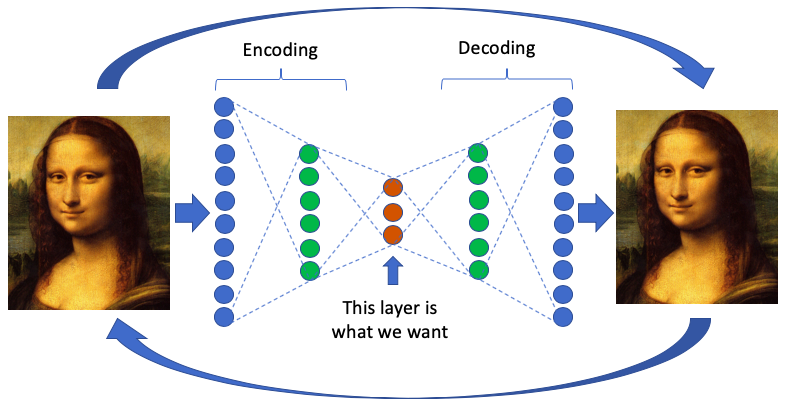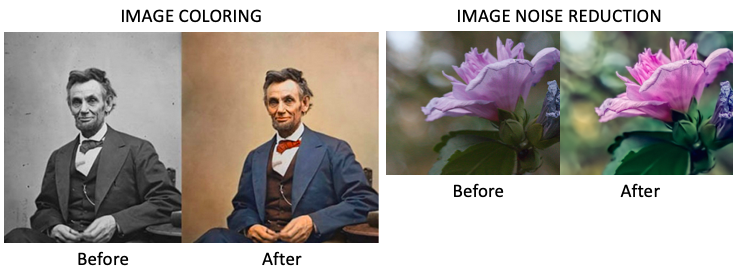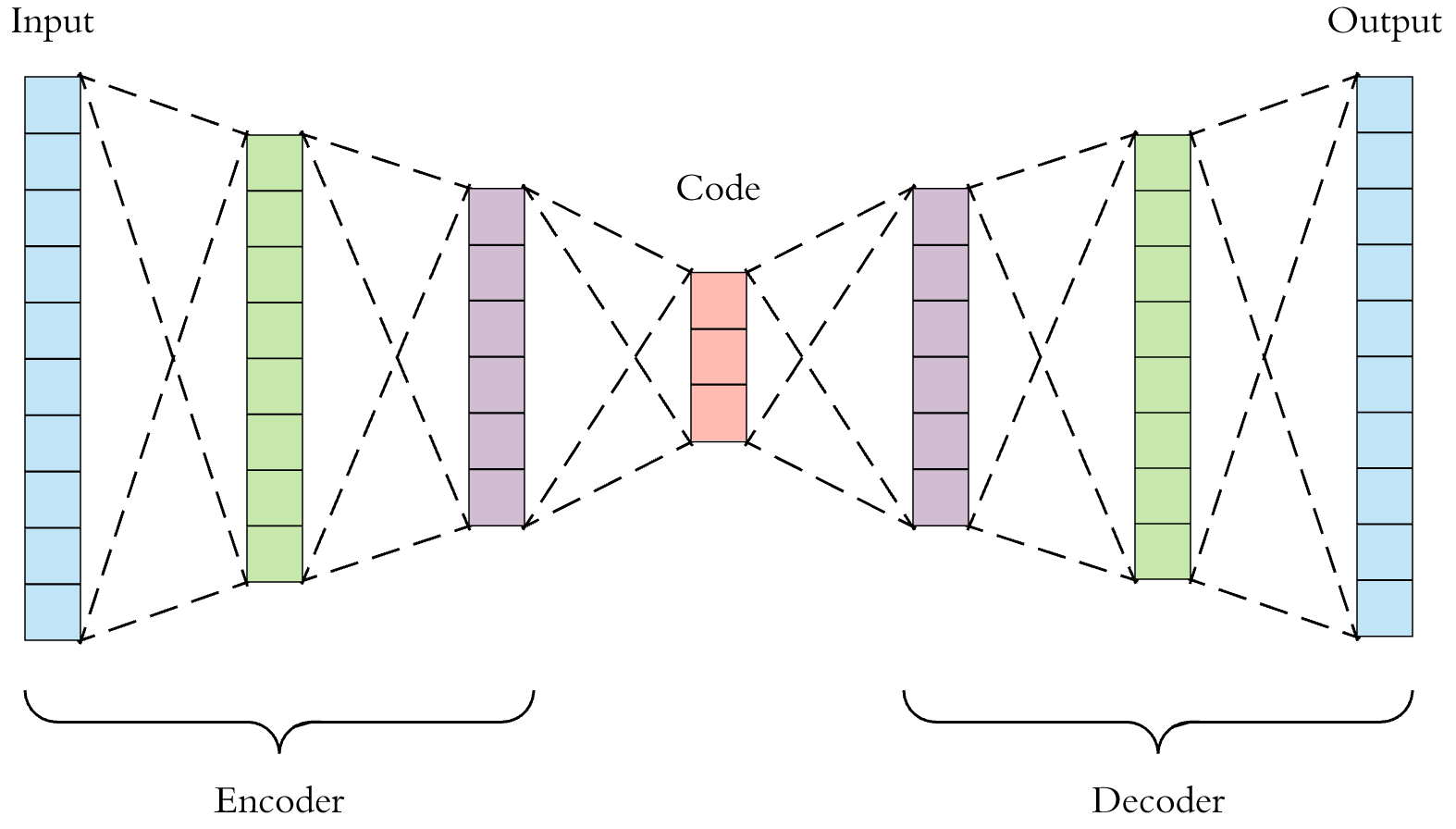##### Autoencoder model and its applications

An autoencoder is a type of neural network that transfers input value to output value, as shown in figure (1). Since the autoencoder model does not …

Feb. 20, 2022 4 minuteAn autoencoder is a type of neural network that transfers input value to output value, as shown in figure (1). Since the autoencoder model does not need a target value (Y), we can call it unsupervised learning.

In the autoencoder model, we are not interested in the output layer. In fact, in this model, hidden layers are essential. If hidden layers are less than input layers, the hidden layers will not extract the necessary information of the input values.Figure 1: Structure of autoencoder model

The hidden layers learn the main patterns of data and remove the noises. In the autoencoder model, the hidden layers have fewer dimensions than the input and output layers.

If the number of neurons in hidden layers is more than neurons in input layers, the neural network will be given too much capacity to learn the data. Sometimes, it may copy the input to the output values, including noises.

Figure (1) also shows the encoding and decoding process. The encoding process compacts the input values to get core layers. The decoding layers rebuild the information to produce the output.## Applications of autoencoder model

1. The early application of autoencoder is dimensionality reduction.
2. The autoencoder models also have broad applications in computer vision and image editing.
3. Autoencoders convert a black-and-white image to a colored one in image coloring.
4. The autoencoder models are used to remove noises.
5. Another application of autoencoder is to compress data like images.

In the autoencoder models, outliers are identified and will not be removed during dimensionality reduction. There are many tools to detect outliers, like PCA. But PCA uses linear algebra to transform.

In contrast, the autoencoder models can perform non-linear transformations with their non-linear activation function and multiplier layers.

Training several layers with an autoencoder is more efficient than training one massive transformation with PCA. The autoencoder technique is the best option when the data is complex and non-linear.## A deep learning model of an autoencoder

The simple form of the encoder is just like the multilayer perceptron, which consists of an input layer, a hidden layer, or an output layer.

A significant difference between the conventional multilayer perceptron neural network and the autoencoder is in the number of nodes in the output layer.

In encoder mode, the output layer contains the same number of nodes as the input layer. Instead of predicting target values ​​according to the output vector, the autoencoder must predict its inputs.

The outline of the learning mechanism in autoencoder is as follows:

1. Calculating the activation functions provided in the hidden and output layers.
2. Finding the deviation between the calculated values ​​with the inputs using the appropriate error function (Loss function).
3. Error propagation to update the weights.
4. Repeating the output until a satisfactory result is reached if the number of nodes in hidden layers is less than the number of input nodes.## A stacked denoising autoencoder model

We explore an original strategy for building deep networks based on stacking layers of denoising autoencoders trained locally to denoise corrupted versions of their inputs.

The resulting algorithm is a straightforward variation on the stacking of ordinary autoencoders.

It is shown on a benchmark of classification problems to yield significantly lower classification error, thus bridging the performance gap with deep belief networks (DBN) and, in several cases, surpassing it.

Higher level representations learned in this purely unsupervised fashion also help boost the performance of subsequent SVM classifiers.

Qualitative experiments show that, contrary to ordinary autoencoders, denoising autoencoders can learn Gabor-like edge detectors from natural image patches and more giant stroke detectors from digit images.

This work establishes the value of using a denoising criterion as a tractable unsupervised objective to guide the learning of useful higher-level representations.## Conclusion

Autoencoders form a fascinating group of neural network architectures with many applications in computer vision, natural language processing, and other fields.

Although there are undoubtedly other classes of models used for representation learning nowadays, such as siamese networks and others, autoencoders remain a good option for various problems.